### Home > A2C > Chapter 11 > Lesson 11.3.1 > Problem11-136

11-136.
1. Given the matrices A, B, and C shown below, calculate each of the following (if possible). Homework Help ✎

 ABBA2A + CAC − A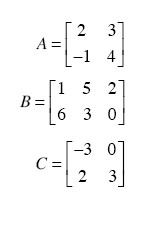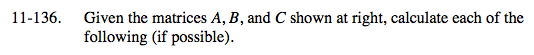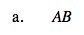Multiply the appropriate rows into the columns and then add.

$\begin{bmatrix}20 & 19 & 4 \\ 23 & 7 & -2 \end{bmatrix}$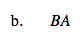Is the number of columns in the first matrix equal to the number or rows in the second matrix?

Not possible.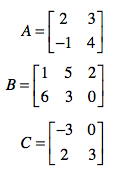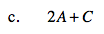$\begin{bmatrix} 1 & 6\\0 & 11 \end{bmatrix}$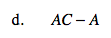$\begin{bmatrix} -2 & 6 \\ 12 & 8 \end{bmatrix}$